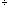Quandaries and Queries please help me work this math problem 38 minus 10 plus 12 divided by 4 times 16 James Hi James, This is somewhat confusing. I expect that you got it from some contest, "The winning contestant must answer a skill testing question". If so then I think that you are to read from left to right and perform the operations as you go. 38 minus 10 is 28 28 plus 12 is 40 40 divided by 4 is 10 10 times 16 is 160 Thus the answer is 160. The confusion is that mathematicians have an order in which they do arithmetic operations. The convention is to perform divisions and multiplications before additions and then subtractions. Hence if the problem had been written using mathematical notation rather than words, 38 - 10 + 12416 I would probably do this as 38 - 10 + 12416 124 = 3 hence 38 - 10 + 316. 316 = 48 hence 38 - 10 + 48 = 76 and get an answer of 76. Harley Go to Math Central